# RBSE Maths Class 9 Chapter 2: Important Questions and Solutions

RBSE Maths Chapter 2 – Number System Class 9 Important questions and solutions are given here. The important questions and solutions of Chapter 2, available at BYJU’S, have stepwise solutions. These solutions will help the students to improve their problem-solving skills and to get full marks for the questions from this chapter.

Chapter 2 of the RBSE Class 9 Maths will help the students to understand rational and irrational numbers. The methods involved to identify the number, whether they are rational or irrational are easy. In this chapter, students will learn how to locate an irrational number on the number line with solved examples.

### RBSE Maths Chapter 2: Exercise 2.1 Textbook Important Questions and Solutions

Question 1: Which of the following is the nature of decimal representation of an irrational number?

1. Non-terminating
2. Terminating
3. Non-terminating recurring
4. Non-terminating non-recurring

Solution:

We know that the decimal representation of an irrational number should be non-terminating non-recurring (repeating), otherwise it will be a rational number.

Question 2: Identify the rational number among the following

1. √255
2. √23
3. 0.3797
4. 1.101001000100001…

Solution:

√255 is not a rational number, because 255 is not a perfect square of any natural number.

√23 is not a rational number, because 23 is not a perfect square of any natural number.

0.3797 is a rational number since it has terminating decimal expansion that means it can be written in the form of p/q where q ≠ 0.

0.3797 = 3797/10000

1.101001000100001… is an irrational number since it has non-terminating, non-repeating decimal expansion.

Question 3: Which of the following is an example of an irrational number?

1. 0.474747..
2. 0.237492371…
3. 36/1000
4. 1.2791

Solution:

0.474747… is a rational number since it has non-terminating repeating decimal expansion.

0.237492731… is an irrational number since it has non-terminating non-repeating decimal expansion.

36/1000 is in the form of p/q where p, q are integers and q ≠ 0. Hence, it is a rational number.

1.2791 is a rational number since it can be written in the form of p/q.

12791/10000

Question 4: The decimal expansion of 32/400 is equal to

1. 0.78
2. 0.80
3. 0.09
4. 0.08

Solution:

32/400 = 8/100 = 0.08

Question 5: Find the rational number that exists between 3 and 4.

1. 7/2
2. 8/3
3. 4/3
4. 11/4

Solution:

7/2 = 3.5

8/3 = 2.666…

4/3 = 1.333…

11/4 = 2.75

From the above, it is clear that 3.5 i.e. 7/2 exists between 3 and 4.

Question 6: Which among the following can be the decimal representation of √5?

1. 2.2360
2. Terminating decimal
3. Non-terminating non-repeating decimal
4. Non-terminating repeating

Solution:

We know that √2, √3, √5… are irrational numbers since their decimal expansion is non-terminating non-repeating. In other words 2, 3, 5, … are not perfect squares and hence, we will not get the exact number under square root.

Question 7: Between two rational numbers there will be

1. no rational number
2. infinite number of rational numbers exist
3. exactly one rational number
4. only one rational number and no irrational numbers

Solution:

There will be an infinite number of rational numbers that exist between two rational numbers.

Question 8: If a number has a terminating decimal, then it is

1. a rational number
2. an integer
3. a whole number
4. a natural number

Solution:

If a number has a terminating decimal, then it is a rational number. Since the decimal representation of a rational number is terminating or non-terminating, recurring.

Question 9: Express the following in the form of p/q where p and q are integers and q ≠ 0.

(i) 0.3 (ii) $$1.\bar{27}$$ (ii) $$1.2\bar{35}$$

Solution:

(i) 0.3 = 3/10

(ii) $$1.\bar{27}$$

Let $$x=1.\bar{27}=1.272727$$ ….(i)

By multiplying (i) with 100,

100x = 127.2727 ….(ii)

(ii) – (i),

100x – x = 127.2727 – 1.272727

99x = 126

x = 126/99

x = 14/11

Therefore, $$1.\bar{27}=\frac{14}{11}$$

(iii) $$1.2\bar{35}$$

Let $$x=1.2\bar{35}=1.2353535$$ ….(i)

To reduce the complexity of simplification we will multiply equ (i) with 10 and the obtained equation with 100.

First multiply (i) with 10,

10x = 12.353535 ….(ii)

Multiplying (ii) with 100,

1000x = 1235.3535 ….(iii)

(iii) – (ii),

1000x – 10x = 1235.3535 – 12.353535

990x = 1223

x = 1223/990

Therefore, $$1.2\bar{35}=\frac{1223}{990}$$

Question 10: Write $$6\frac{1}{8}$$ in decimal form and specify the type of decimal representation.

Solution:

$$6\frac{1}{8}$$

= (6 × 8 + 1)/8

= 49/8

Using long division method,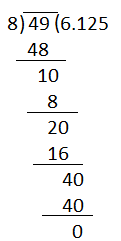49/8 = 6.125

Therefore, the decimal representation is terminating.

### RBSE Maths Chapter 2: Exercise 2.2 Textbook Important Questions and Solutions

Question 11: Add (3√2 + 5√3) and (√2 – √3)

Solution:

(3√2 + 5√3) + (√2 – √3)

= (3 + 1)√2 + (5 – 1)√3

= 4√2 + 4√3

= 4(√2 + √3)

Question 12: Multiply 5√7 and 12√7.

Solution:

5√7 × 12√7 = (5 × 12) (√7 × √7)

= 60 × 7

= 420

Question 13: Rationalise the denominator of 1/(2 – √3).

Solution:

1/(2 – √3) = [1/ (2 – √3)] × [(2 + √3)/ (2 + √3)]

= (2 + √3)/ [22 – (√3)2]

= (2 + √3)/ (4 – 3)

= (2 + √3)/ 1

= 2 + √3

Question 14: Classify the following numbers as rational or irrational:

(i) 2 – √5 (ii) 1/√3 (iii) (3 + √23) – √23 (iv) 5π

Solution:

2 – √5 is an irrational number since √5 is irrational.

1/√3 is an irrational number since √3 is irrational.

(3 + √23) – √23 = 3, it is a rational number.

5π is an irrational number since π is irrational. (value of π has determined exactly)

Question 15: Rationalise the denominator of the following number.

1/(5 + 3√7)

Solution:

$$\frac{1}{5+3\sqrt{7}}=\frac{1}{5+3\sqrt{7}}\times \frac{5-3\sqrt{7}}{5-3\sqrt{7}}$$ $$=\frac{5-3\sqrt{7}}{5^2-(3\sqrt{7})^2}$$ $$=\frac{5-3\sqrt{7}}{25-(9\times 7)}\\=\frac{5-3\sqrt{7}}{25-63}\\=\frac{5-3\sqrt{7}}{-38)}\\=\frac{-5}{38}+\frac{3\sqrt7}{38}$$

Question 16: If $$\frac{3+2\sqrt 2}{3-\sqrt 2}=a+b\sqrt{2}$$ then find the value of a and b, where a and b are real numbers.

Solution:

Given.

$$\frac{3+2\sqrt 2}{3-\sqrt 2}=a+b\sqrt{2}$$

Consider LHS of the given equation,

$$\frac{3+2\sqrt 2}{3-\sqrt 2}=\frac{3+2\sqrt 2}{3-\sqrt 2}\times \frac{3+\sqrt 2}{3+\sqrt 2} \\=\frac{(3+2\sqrt 2)(3+\sqrt 2)}{(3)^2-(\sqrt 2)^2)}$$ $$=\frac{9+6\sqrt{2}+3\sqrt{2}+4}{9-2}$$ $$=\frac{13+9\sqrt{2}}{7}\\=\frac{13}{7}+\frac{9\sqrt2}{7}$$

Now comparing the like term of LHS and RHS,

a = 13/7 and b = (9√2)/7

Question 17: Divide (7√13)/ (2√13).

Solution:

(7√13)/ (2√13)

By cancelling √13 in both numerator and denominator,

= 7/2

Or

= 3.5

Question 18: What is the value of (√243)/√3 ?

Solution:

(√243)/ √3

= [√(9 × 9 × 3)] / √3

= (9 √3)/ √3

By cancelling √3 in both numerator and denominator,

= 9

Question 19: Show √3 on the number line.

Solution:

Take two triangles namely OAB and OBD on the number line with two of the sides of triangle OAB equal to unity i.e.1.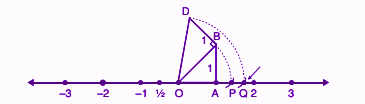In the above figure, AB is perpendicular to OA.

By Pythagoras theorem,

OB2 = OA2 + AB2

OB2 = 12 + 12

OB2 = 1 + 1

OB2 = 2

OB = √2

Triangle OBD is a right triangle.

Again by Pythagoras theorem,

OD2 = BD2 + OB2

OD2 = 12 + (√2)2

OD2 = 1 + 2

OD2 = 3

OD = √3

Taking O as the centre and with the radius OD, draw an arc which intersects the number line at Q where Q corresponds to √3.

Question 20: Rationalise the denominator of 1/(2√7 – 5).

Solution:

1/(2√7 – 5) = [1/ (2√7 – 5)] × [(2√7 + 5)/ (2√7 + 5)]

= (2√7 + 5)/ [(2√7)2 – 52]

= (√2√7 + 5)/ [4(7) – 25]

= (2√7 + 5)/ (28 – 25)

= (2√7 + 5)/3

### RBSE Maths Chapter 2: Exercise 2.2 Textbook Important Questions and Solutions

Question 21: Simplify the following:

(i) (64) (ii) (125)

Solution:

(i) (64)

Since the power is ⅙, we should write 64 in terms of power 6.

26 = 64

(26) = 26 × ⅙ = 2

(ii) (125)

Since the power is ⅓ , we should write 125 in terms of power 3.

125 = 53

(53) = 53 × ⅓ = 5

Question 22: Find the value of (4)3/2.

Solution:

(4)3/2

Since the denominator of power is 2, we should write 4 in terms of power 2

22 = 4

(2.2)3/2 = 2(2 × 3/2)

= 23

= 8

Question 23: What is the simplest form of (32).

Solution:

(32)

Since the denominator of power is 5, we should write 32 in terms of power 5.

25 = 32

(32) = (25)

= 2(5 × 2/5)

= 22

= 4

Question 24: Simplify: 21/3 . 22/7

Solution:

21/3 . 22/7

Bases are equal, hence add the powers.

= 2(1/3 + 2/7)

= 2(7 + 6)/21

= 213/21

Question 25: If $$[\frac{3}{5}]^x[\frac{5}{3}]^{2x}=\frac{125}{27}$$, then find the value of x.

Solution:

Given,

$$[\frac{3}{5}]^x[\frac{5}{3}]^{2x}=\frac{125}{27}$$

Using the formula $$[\frac{a}{b}]^n = [\frac{b}{a}]^{-n}$$ $$[\frac{5}{3}]^{-x}[\frac{5}{3}]^{2x}=\frac{5^3}{3^3}$$

Here bases of the LHS are equal so we are adding the powers.

$$[\frac{5}{3}]^{-x+2x}=[\frac{5}{3}]^3$$

Now bases of both LHS and RHS are equal and hence equating the powers,

-x + 2x = 3

x = 3

Therefore, the value of x is 3.

Question 26: Simplify (17)¼ . (17)¾

Solution:

(17)¼ . (17)¾

Since bases are equal, we are adding the powers of 17

17(¼ + ¾)

= 17(1 + 3)/4

= 174/4

= 171

= 17

Question 27: Calculate the value of (512)2/9.

Solution:

(512)2/9

Here the denominator of the power is 9 and hence, we should write 512 in terms of power 9.

29 = 512

Thus,

(29)2/9 = 2(2 × 2/9)

= 22

= 4

Question 28: Simplify: $$[\frac{1}{5^7}]^{3}$$

Solution:

$$[\frac{1}{5^7}]^{3}$$ $$=[\frac{1}{(5^7)^3}]$$ $$=[\frac{1}{5^{21}}]$$

= 5-21

### RBSE Maths Chapter 1: Additional Important Questions and Solutions

Question 1: Express 0.13 in the form of p/q, where p and q are integers and q ≠ 0.

Solution:

0.13

There are two digits after the decimal point.

0.13 = 13/100, which is of the form p/q with p = 13 and q = 100.

Question 2: Rationalise the denominator of 1/(7 + 3√2).

Solution:

1/(7 + 3√2) = [1/ (7 + 3√2)] × [(7 – 3√2)/ (7 – 3√2)]

= (7 – 3√2)/ [72 – (3√2)2]

= (7 – 3√2)/ (49 – 18)

= (7 – 3√2)/ 31

Question 3: Rationalise: 1/(2 + √3)

Solution:

1/(2 + √3) = [1/ (2 + √3)] × [(2 – √3)/ (2 – √3)]

= (2 – √3)/ [22 – (√3)2]

= (2 – √3)/ (4 – 3)

= (2 – √3)/ 1

= 2 – √3

Question 4: Rationalise the denominator of 1/ (√5 – 2).

Solution:

1/(√5 – 2) = [1/ (√5 – 2)] × [(√5 + 2)/ (√5 + 2)]

= (√5 + 2)/ [(√5)2 – 22]

= (√5 + 2)/ (5 – 4)

= (√5 + 2)/ 1

= √5 + 2

Question 5: Find four rational numbers between 3 and 4.

Solution:

There are an infinite number of rational numbers between 3 and 4.

Now we need to find 4 rational numbers between 3 and 4.

We will multiply both the number 3 and 4 with 5 (i.e. 4 + 1 = 5) or any number greater than 4.

3 × 5/5 = 15/5

4 × 5/5 = 20/5

Thus, the rational numbers between 15/5 and 20/5 will be rational and fall between 3 and 4.

Therefore, 16/5, 17/5, 18/5, and 19/5 are the four rational numbers between 3 and 4.

Question 6: Simplify: (216)

Solution:

(216)

Here, the denominator of the power is 3. So we need to write 216 in terms of power 3.

63 = 216

(216) = (63)

= 6(3 × ⅓)

= 61

= 6

Question 7: Rationalise the denominator of 1/ (√7 + 2).

Solution:

1/(√7 + 2) = [1/ (√7 + 2)] × [(√7 – 2)/ (√7 – 2)]

= (√7 – 2)/ [(√7)2 – 22]

= (√7 – 2)/ (7 – 4)

= (√7 – 2)/ 3

Question 8: Express $$1.4\bar{75}$$ in the form of p/q where p and q are integers and q ≠ 0.

Solution:

Let $$x=1.4\bar{75}=1.47575$$….(i)

Multiplying equ (i) with 10,

10x = 14.7575….(ii)

Again multiplying (ii) with 100,

1000x = 1475.75

(iii) – (ii),

1000x – 10x = 1475.75 – 14.7575

990x = 1461

x = 1461/990

Which is of the form p/q and q ≠ 0

Question 9: What is the nature of decimal representation of a rational number?

Solution:

A rational has a decimal representation which is terminating or non-terminating repeating. For example, 1.23, 0.345, 0.6725725…., 3.11111…. and son on.

Question 10: Find the value of √128 ÷ √2.

Solution:

√128 ÷ √2

Using the formula $$\sqrt{a}\div \sqrt{b}=\sqrt{\frac{a}{b}}$$

= √(128/2)

= √64

= √(8 × 8)

= 8

Question 11: Write $$5.\bar{4}$$ in the form of p/q where p, q are integers and q ≠ 0.

Solution:

Let $$x=5.\bar{4}=5.4444$$ ….(i)

Multiplying equation (i) with 10,

10x = 54.444 ….(ii)

(ii) – (i),

10x – x = 54.444 – 5.4444

9x = 49

x = 49/9

Question 12: Is π an irrational number? Explain.

Solution:

Yes, π is an irrational number.

We know that the ratio of the circumference of a circle to its diameter is equal to π. And the value of π is approximately equal to 22/7 or 3.1428571… Since there is no exact value for π we can say that it is irrational.

Question 13: Simplify the following:

(5 + √4)(5 – √4)

Solution:

(5 + √4)(5 – √4)

Using the identity (a + b)(a – b) = a2 – b2

= (5)2 – (√4)2

= 25 – 4

= 21

Question 14: Show √2 on the number line.

Solution:

Draw a square OABC with unit length on the side.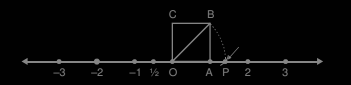In the above figure, AB is perpendicular to OA.

By Pythagoras theorem,

OB2 = OA2 + AB2

OB2 = 12 + 12

OB2 = 1 + 1

OB2 = 2

OB = √2

Taking the distance of OB in a compass and with centre O, draw an arc on the number line that intersects it at the point P. Hence, this is point P on the number line corresponding to √2 .

Question 15: Find an irrational number between 4/7 and 5/7.

Solution:

We know that,

4/7 = 0.57142857142…..

5/7 = 0.7142857142….

Thus, we can write an infinite number of irrational numbers between 4/7 and 5/7, which have non-terminating non-repeating decimal expansion.

For example, 0.67142867…., 0.606006000600…

Question 16: Express 115/4 in decimal form and write the type of decimal representation.

Solution: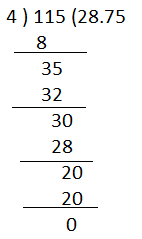115/4 = 28.75

It is a terminating decimal expansion.

Question 17: Which of the following is different from the other numbers?

1. √7
2. √16
3. √13
4. √15

Solution:

Among 7, 16, 13, and 15, only 16 is the perfect square number. Hence, √16 = 4, is a rational number whereas others are irrational numbers.

Question 18: Simplify the following expression:

(√11 – 2√3)2

Solution:

(√11 – 2√3)2

Using the formula (a – b)2 = a2 – 2ab + b2

= (√11)2 – 2(√11)(2√3) + (2√3)2

= 11 – 4√(11 × 3) + (4 × 3)

= 11 – 4√33 + 12

= 1 – 4√33

Question 19: Convert 300/130 into decimal form and write the type of decimal expansion.

Solution:

300/130 = 30/13

Using the long division method,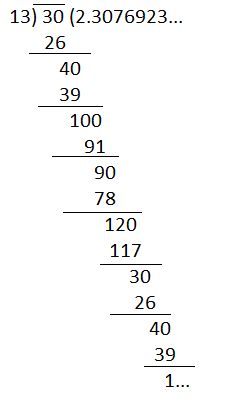300/130 = 2.307692307….

Hence, the decimal expansion of 300/130 is non-terminating repeating.

Question 20: Write any four decimal numbers whose decimal representation is non-terminating non-repeating.

Solution:

There exist an infinite number of decimal numbers whose decimal expansion is non-terminating non-repeating. Some of them are:

0.2010200210….

1.456217010130217….

9.303003000300003…

5.237547587458….

Question 21: Simplify the following:

(i) 51/2. 61/2 (ii) $$=\frac{16^{\frac{1}{2}}}{16^{\frac{1}{4}}}$$

Solution:

(i) 51/2 . 61/2

Using the formula am . bm = (ab)m

= (5 × 6)1/2

= (30)1/2

= √30

(ii) $$=\frac{16^{\frac{1}{2}}}{16^{\frac{1}{4}}}$$

Using the formula am/an = am – n

= 16(½ – ¼)

= 16(2 – 1)/4

= 161/4

This can be further simplified as below.

= (24)¼

= 2(4 × ¼)

= 2

Question 22: If (1/5)8 – 4x = √5, then find the value of x.

Solution:

(1/5)8 – 4x = √5

Using the formula (1/a)n = a-n,

5-(8 – 4x) = 51/2

Bases are equal on both sides. Hence, equating the powers,

-(8 – 4x) = ½

4x – 8 = ½

4x = 8 + ½

4x = (16 + 1)/2

4x = 17/2

x = 17/8